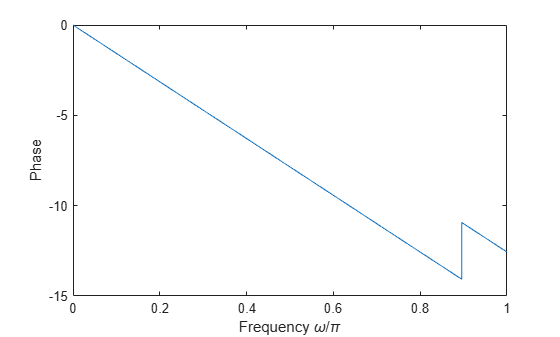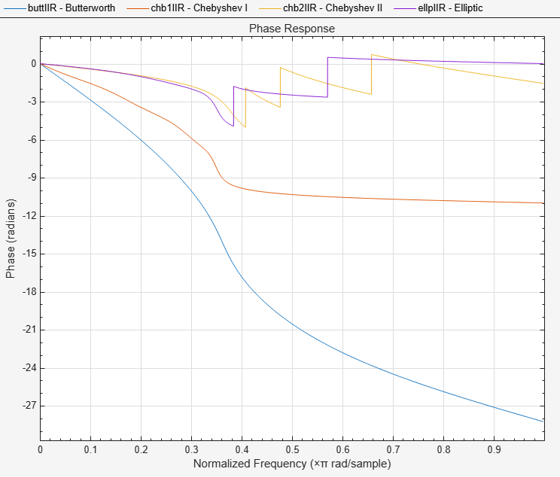# islinphase

Verify that discrete-time filter System object is linear phase

## Syntax

flag = islinphase(sysobj)
flag = islinphase(sysobj,tol)
flag = islinphase(___,'Arithmetic',arithType)

## Description

example

flag = islinphase(sysobj) returns true if the filter System object™ has linear phase.
flag = islinphase(sysobj,tol) uses the tolerance tol to determine when two numbers are close enough to be considered equal. If not specified, tol defaults to eps^(2/3).
flag = islinphase(___,'Arithmetic',arithType) analyzes the filter System object based on the arithmetic specified in the arithType input using either of the previous syntaxes.For more input options, see islinphase in Signal Processing Toolbox™.

## Examples

collapse all

Use the window method to design a 10th order lowpass FIR filter with the normalized cutoff frequency of 0.55. Verify that the filter has linear phase.

firSpecs = fdesign.lowpass('N,Fc',10,0.55); lpFIR = design(firSpecs,'window','SystemObject',true); flag = islinphase(lpFIR)
flag = logical 1 

Plot the phase response of the filter and verify that it is linear.

[phs,w] = phasez(lpFIR); plot(w/pi,phs) xlabel('Frequency \omega/\pi') ylabel('Phase')IIR filters in general do not have linear phase. Verify this by constructing Butterworth, Chebyshev, and elliptic filters with similar specifications. Set the passband frequency to 0.35, stopband frequency to 0.4, passband ripple to 1 dB, and stopband attenuation to 20 dB.

Wp = 0.35; Wst = 0.4; atten = 20; rippl = 1; buttSpecs = fdesign.lowpass('Fp,Fst,Ap,Ast',Wp,Wst,rippl,atten); buttIIR = design(buttSpecs,'butter','SystemObject',true); chb1Specs = fdesign.lowpass('Fp,Fst,Ap,Ast',Wp,Wst,rippl,atten); chb1IIR = design(chb1Specs,'cheby1','SystemObject',true); chb2Specs = fdesign.lowpass('Fp,Fst,Ap,Ast',Wp,Wst,rippl,atten); chb2IIR = design(chb2Specs,'cheby2','SystemObject',true); ellpSpecs = fdesign.lowpass('Fp,Fst,Ap,Ast',Wp,Wst,rippl,atten); ellpIIR = design(ellpSpecs,'ellip','SystemObject',true);

Plot the phase responses of the filters. Determine whether they have linear phase.

fv = fvtool(buttIIR,chb1IIR,chb2IIR,ellpIIR,'Analysis','phase'); legend(fv,'Butterworth','Chebyshev I','Chebyshev II','Elliptic')phs = [islinphase(buttIIR) islinphase(chb1IIR) ... islinphase(chb2IIR) islinphase(ellpIIR)]
phs = 1x4 logical array 0 0 0 0 

## Input Arguments

collapse all

Tolerance value to determine when two numbers are close enough to be considered equal, specified as a positive scalar. If not specified, tol, defaults to eps^(2/3).

Arithmetic used in the filter analysis, specified as 'double', 'single', or 'Fixed'. When the arithmetic input is not specified and the filter System object is unlocked, the analysis tool assumes a double-precision filter. When the arithmetic input is not specified and the System object is locked, the function performs the analysis based on the data type of the locked input.

The 'Fixed' value applies to filter System objects with fixed-point properties only.

When the 'Arithmetic' input argument is specified as 'Fixed' and the filter object has the data type of the coefficients set to 'Same word length as input', the arithmetic analysis depends on whether the System object is unlocked or locked.

• unlocked –– The analysis object function cannot determine the coefficients data type. The function assumes that the coefficients data type is signed, has a 16-bit word length, and is auto scaled. The function performs fixed-point analysis based on this assumption.

• locked –– When the input data type is 'double' or 'single', the analysis object function cannot determine the coefficients data type. The function assumes that the data type of the coefficients is signed, has a 16-bit word length, and is auto scaled. The function performs fixed-point analysis based on this assumption.

To check if the System object is locked or unlocked, use the isLocked function.

When the arithmetic input is specified as 'Fixed' and the filter object has the data type of the coefficients set to a custom numeric type, the object function performs fixed-point analysis based on the custom numeric data type.

## Output Arguments

collapse all

Flag to determine if the filter has linear phase, returned as a logical:

• 1 –– Filter has linear phase.

• 0 –– Filter has nonlinear phase.

Data Types: logical